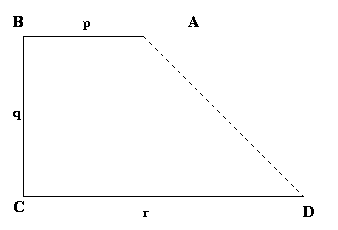#### You may also like### Two Cubes

Two cubes, each with integral side lengths, have a combined volume equal to the total of the lengths of their edges. How big are the cubes? [If you find a result by 'trial and error' you'll need to prove you have found all possible solutions.]### Rationals Between...

What fractions can you find between the square roots of 65 and 67?### Square Mean

Is the mean of the squares of two numbers greater than, or less than, the square of their means?

# Biggest Enclosure

##### Age 14 to 16Challenge LevelShaun of Nottingham High School and also Ben and Thomas sent in good solutions to this problem. Here is Shaun's solution. There are three possibilities we must consider, in each one the 'middle' fence (vertical in the diagram ) is one of $p$, $q$ or $r$. Interchanging the horizontal fences either side makes no difference to the area of the shape, it is just being flipped vertically. Remember the area of a trapezium can be expressed as ${1\over 2}(a+b)h$, where $a$ and $b$ are parallel sides, and $h$ the distance between them.
This means we can express the areas of the three possible shapes as:

$$\frac {1}{2}(p+q)r, \frac {1}{2}(q+r)p, \frac {1}{2}(r+p)q.$$

Let's compare the first two. As $qr> qp$ we have $qr + pr > qp + pr$ and so

$${1\over 2}(p+q)r > {1\over 2}(q+r)p.$$

I hope you can see an identical argument can be used to compare the other pairings of expressions for area, and we end up with:

$${1\over 2}(q+p)r > {1\over 2}(p+r)q > {1\over 2}(r+q)p.$$

So the fences should be arranged with $r$ being the middle one in order to enclose the maximum area.